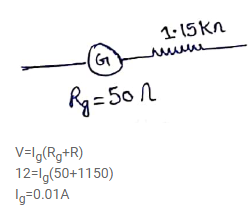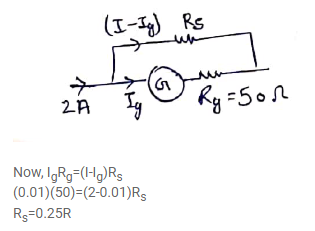# A voltmeter coil has resistance 50.0Ω and resistor of 1.15kΩ

Question:

A voltmeter coil has resistance $50.0 \Omega$ and resistor of $1.15 \mathrm{k} \Omega$ is connected in series. It can read potential differences up to 12 volts. If this same coil is used to construct an ammeter which can measure currents up to $2.0 \mathrm{~A}$, what should be the resistance of the shunt used?

Solution: